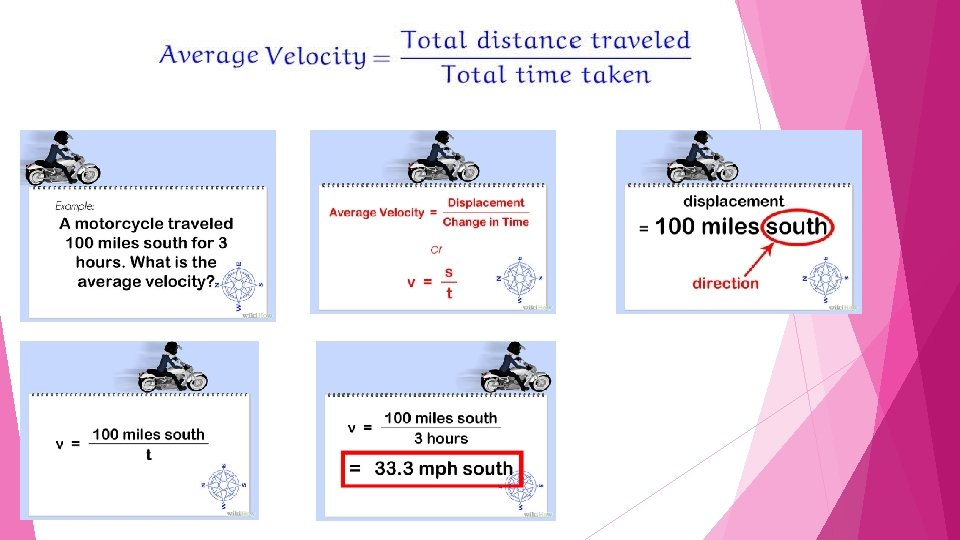# Motion Speed velocity and acceleration LESSON Speed and

• Slides: 22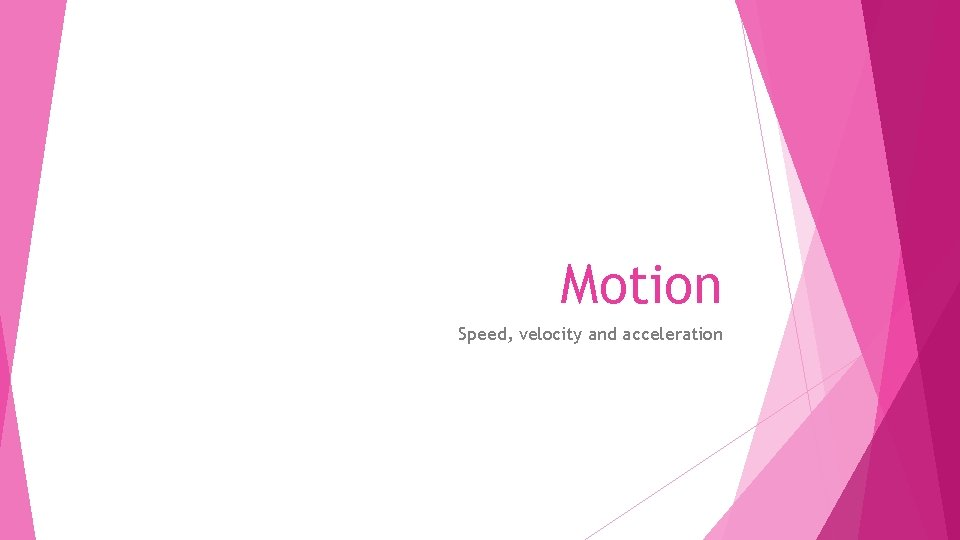Motion Speed, velocity and acceleration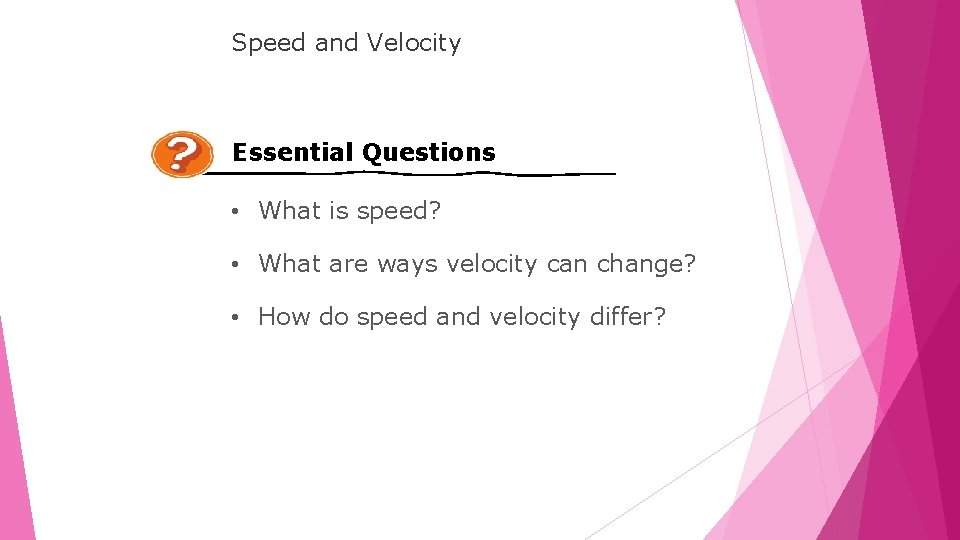LESSON Speed and Velocity Essential Questions • What is speed? • What are ways velocity can change? • How do speed and velocity differ?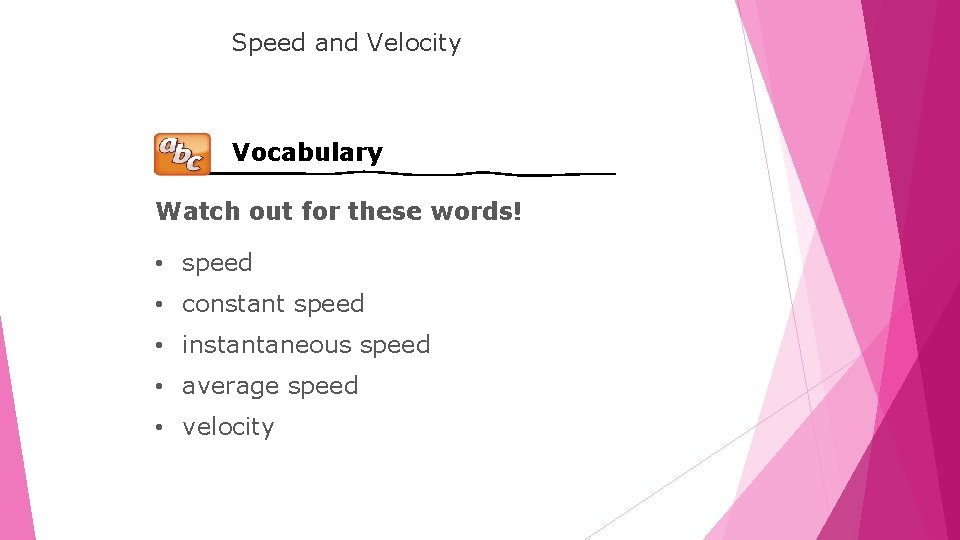LESSON Speed and Velocity Vocabulary Watch out for these words! • speed • constant speed • instantaneous speed • average speed • velocity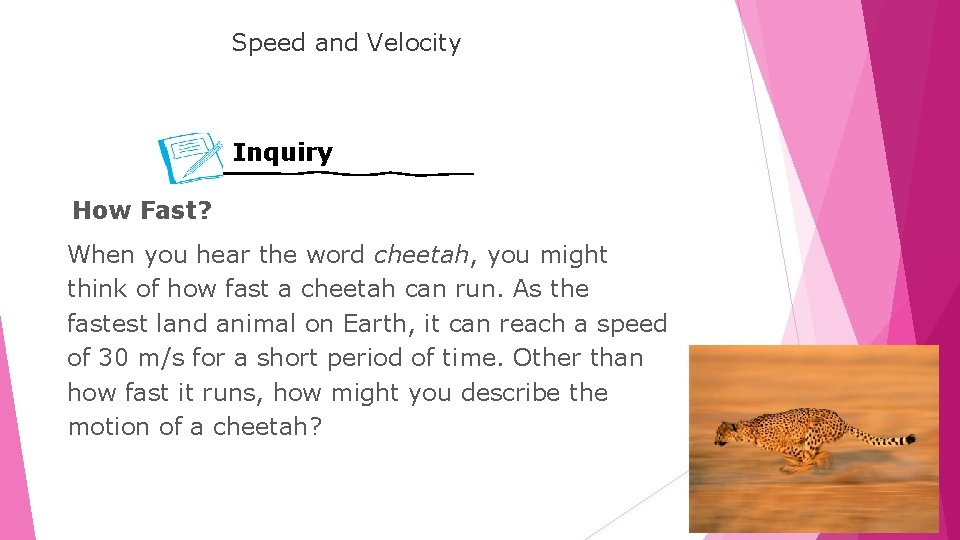LESSON Speed and Velocity Inquiry How Fast? When you hear the word cheetah, you might think of how fast a cheetah can run. As the fastest land animal on Earth, it can reach a speed of 30 m/s for a short period of time. Other than how fast it runs, how might you describe the motion of a cheetah?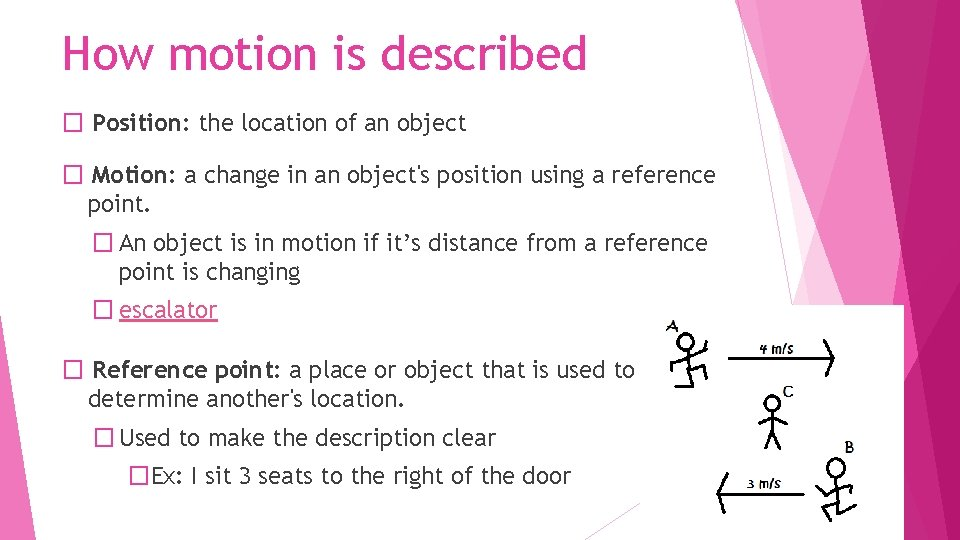How motion is described � Position: the location of an object � Motion: a change in an object's position using a reference point. � An object is in motion if it’s distance from a reference point is changing � escalator � Reference point: a place or object that is used to determine another's location. � Used to make the description clear �Ex: I sit 3 seats to the right of the doorHow is speed described? � Speed: the measure of how quickly distance changes with time (How fast the object goes in a certain amount of time) � Unit of measurement: m/s � Average speed: average speed an object goes including the times speed increased and decreased. � Instantaneous speed: speed of an object at a specific moment in time � Can be different from average speed.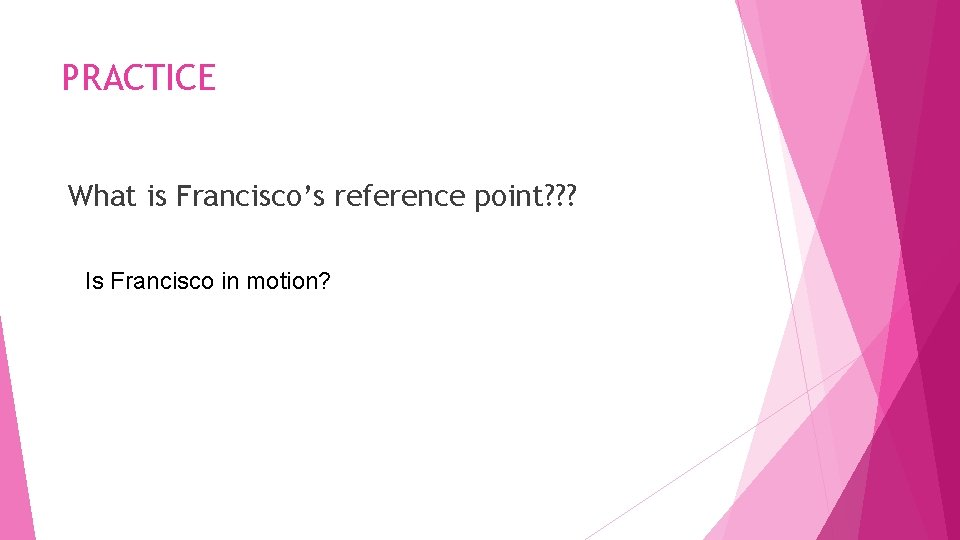PRACTICE What is Francisco’s reference point? ? ? Is Francisco in motion?How is velocity described? � Velocity: speed and direction of a moving object � Represent velocity: arrows � Length indicates speed �Longer = greater speed � Direction �Pointing showing motion or path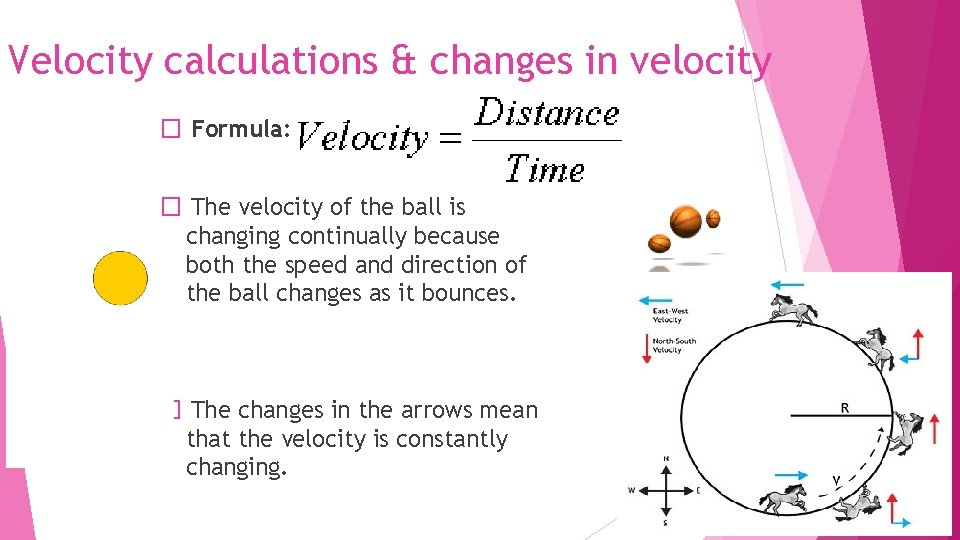Velocity calculations & changes in velocity � Formula: � The velocity of the ball is changing continually because both the speed and direction of the ball changes as it bounces. � The changes in the arrows mean that the velocity is constantly changing.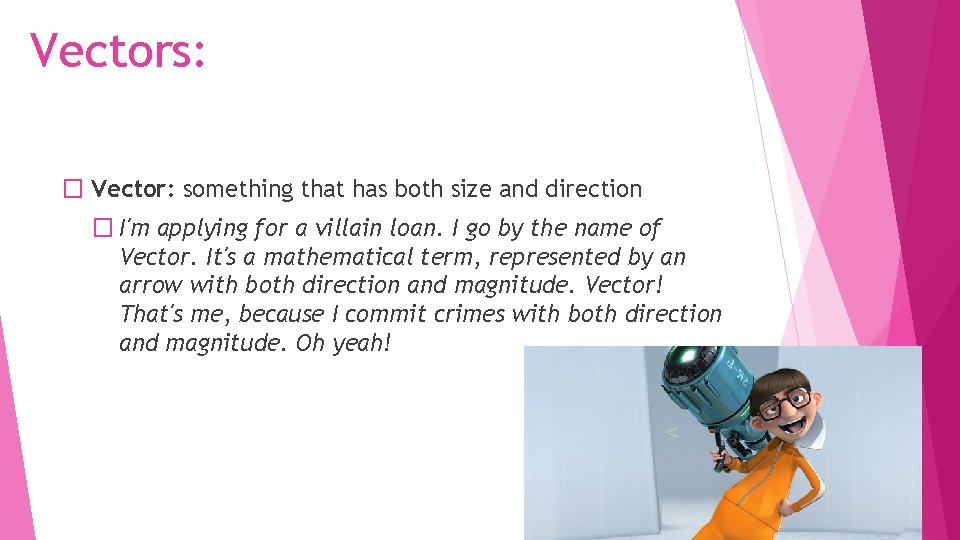Vectors: � Vector: something that has both size and direction � I'm applying for a villain loan. I go by the name of Vector. It's a mathematical term, represented by an arrow with both direction and magnitude. Vector! That's me, because I commit crimes with both direction and magnitude. Oh yeah!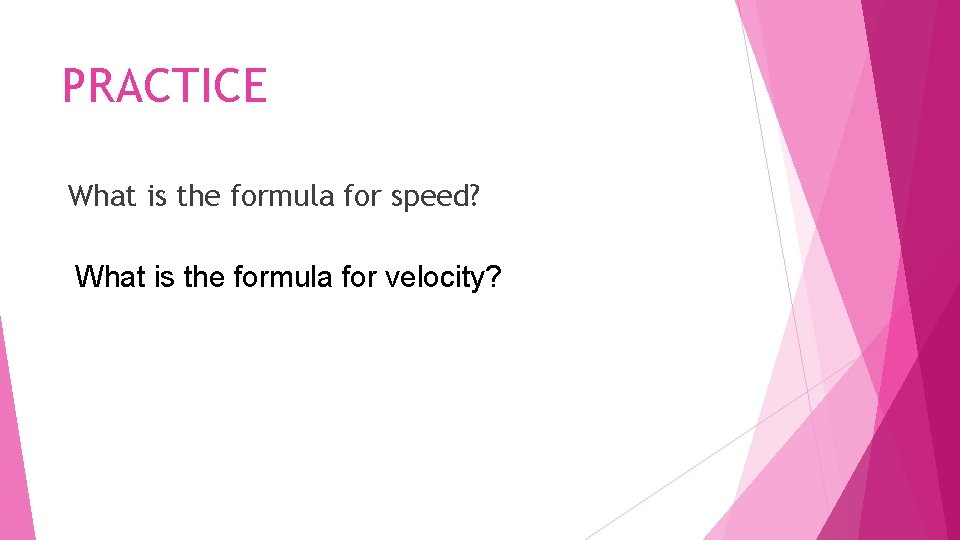PRACTICE What is the formula for speed? What is the formula for velocity?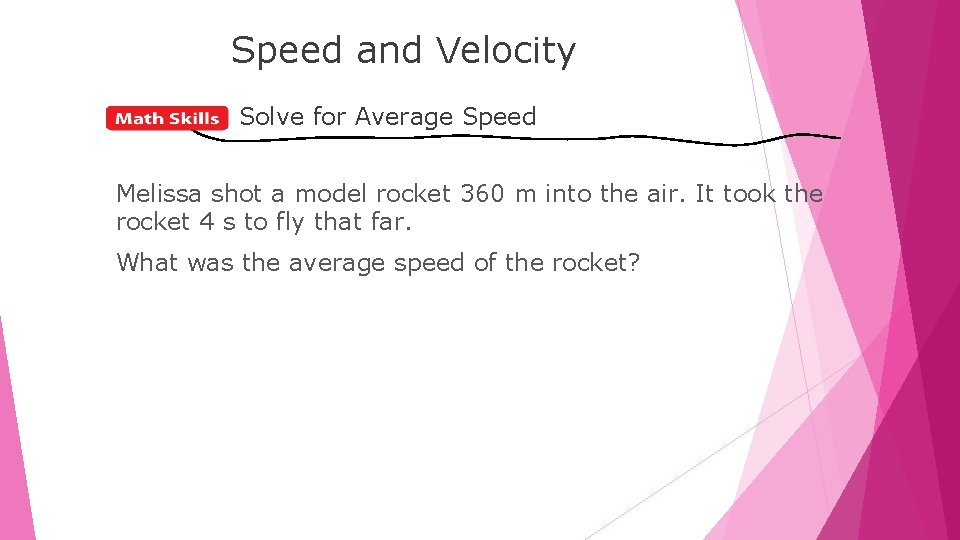LESSON Speed and Velocity Solve for Average Speed Melissa shot a model rocket 360 m into the air. It took the rocket 4 s to fly that far. What was the average speed of the rocket?LESSON Speed and Velocity Solve for Average Speed, continued STEP 1 This is what you know: distance: d = 360 m STEP 2 STEP 3 and time: t = 4 s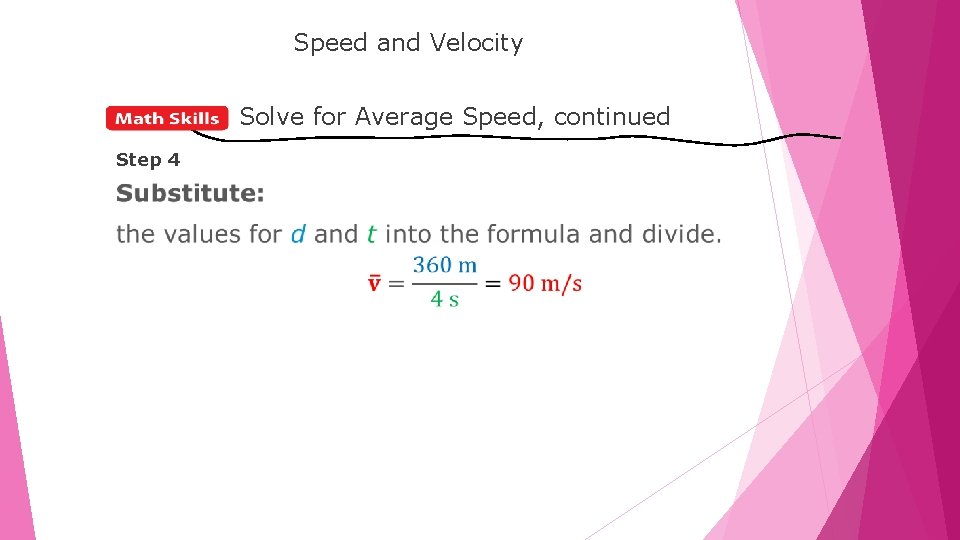LESSON Speed and Velocity Solve for Average Speed, continued Step 4How is acceleration described? � Acceleration is a measure of how quickly velocity changes � Occurs when there is a change is speed, change is direction or bothAcceleration and Velocity � Acceleration is a change in velocity � When velocity is not changing, acceleration is 0. � Ex: a car driving down a hill at a constant speed and direction (constant velocity), has no acceleration.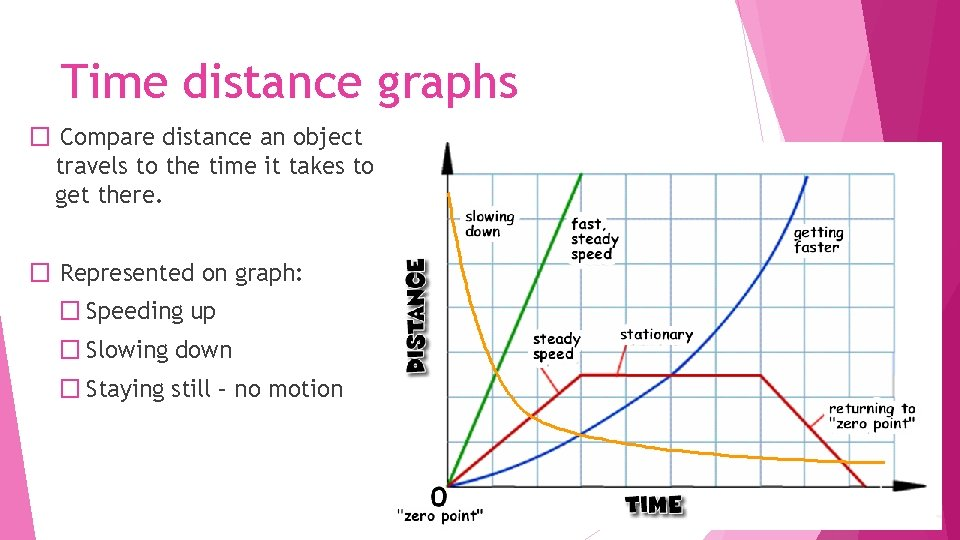Time distance graphs � Compare distance an object travels to the time it takes to get there. � Represented on graph: � Speeding up � Slowing down � Staying still – no motion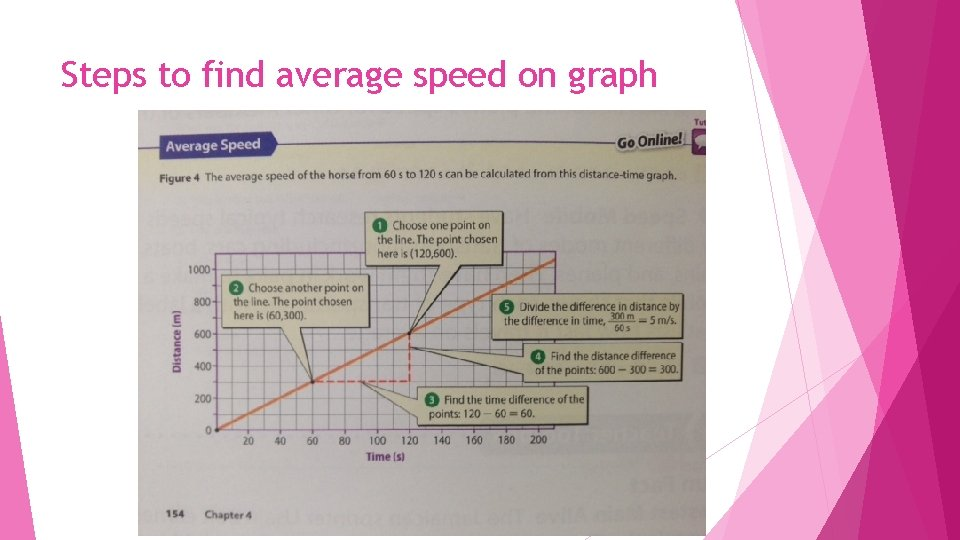Steps to find average speed on graphLESSON Speed and Velocity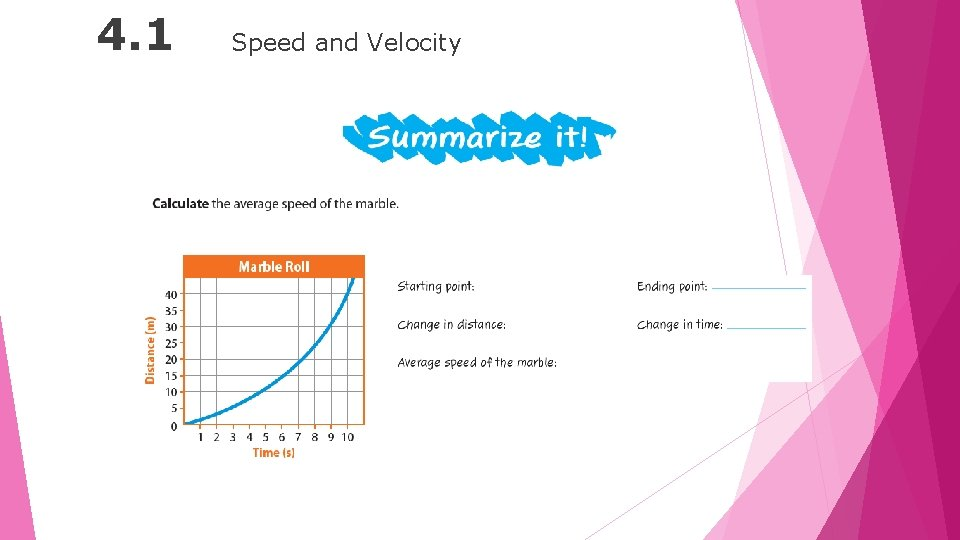LESSON 4. 1 Speed and Velocity